﻿ Robust Lead Compensator Design for an Electromechanical Actuator Based on H∞ Theory

Robust Lead Compensator Design for an Electromechanical Actuator Based on H∞ Theory

Rafik Salloum, Mohammad Reza Arvan, Bijan MoaveniOPEN ACCESSPEER-REVIEWED

Robust Lead Compensator Design for an Electromechanical Actuator Based on H∞ Theory

Rafik Salloum1,, Mohammad Reza Arvan1, Bijan Moaveni2

1Faculty of Electrical Engineering, Malek-Ashtar University of Technology (MUT), 15875-1774, Tehran, Iran

2School of Railway Engineering, Iran University of Science and Technology (IUST), 16846-13114, Tehran, Iran

Abstract

In this paper, we design a robust lead compensator for a real Electromechanical Actuator (EMA) harmonic drive by introducing an approach based on H∞ control theory. Here, we address three main topics; experimental identification, uncertainty modelling, and robust control design for a real EMA harmonic drive system. This method verifies good tradeoff between the powerful H∞ controller and the unique features of compensators, such as: simplicity, low cost and easy implementation. The H∞ controller and the extracted compensator are almost identical within the EMA bandwidth range. Simulation and test results prove the effectiveness of the proposed approach and the superiority of the performance of the designed robust EMA with lead compensator based on H∞ controller over the original EMA; this preference is pertaining to its robustness to parametric uncertainties and high performance.

At a glance: Figures

1234
Prev Next

• Salloum, Rafik, Mohammad Reza Arvan, and Bijan Moaveni. "Robust Lead Compensator Design for an Electromechanical Actuator Based on H∞ Theory." Automatic Control and Information Sciences 2.3 (2014): 53-58.
• Salloum, R. , Arvan, M. R. , & Moaveni, B. (2014). Robust Lead Compensator Design for an Electromechanical Actuator Based on H∞ Theory. Automatic Control and Information Sciences, 2(3), 53-58.
• Salloum, Rafik, Mohammad Reza Arvan, and Bijan Moaveni. "Robust Lead Compensator Design for an Electromechanical Actuator Based on H∞ Theory." Automatic Control and Information Sciences 2, no. 3 (2014): 53-58.

 Import into BibTeX Import into EndNote Import into RefMan Import into RefWorks

1. Introduction

Electromechanical actuators (EMA) are finding increasing use in the robotic and aerospace applications, since they have attractive characteristics such as: simplicity, reliability, low cost, good dynamic characteristics, and easy control [1, 2].

Modelling and identification of the plant properly is the most effective step in control system design procedure. A good model is the simplest model that best explains the dynamics and successfully simulates or predicts the output for different inputs . However, EMA modeling is subjected to uncertainty due to several reasons including operating point changes, perpetual parametric variations because of temperature changes, aging, unmodelled dynamics, and asymmetric behaviour. Consequently, the desired EMA's performance will be unachievable and, in some cases, its stability may be lost. Usually, based on experience, this problem will not be solved by using the conventional controllers; instead robust controllers with regard to the uncertainties in the model are needed to obtain the desired performance and stabilization demands in dealing with dynamic uncertainties .

Much effort is devoted to design robust controllers for uncertain systems based on different robust design methods known in literature such as Kharitonov's theorem, small gain theorem, H, and Quantitative Feedback Theory (QFT) [5, 6, 7]. Computational methods for determining the set of all stabilizing controllers, of a given order and structure, for linear time-invariant delay free systems are reported in [8, 9]. A graphical design method of tuning the PI and PD controllers achieving gain and phase margins is developed in [10, 11]. Besides, in , the Kharitonov theorem is exploited for characterizing all PID controllers that stabilize an uncertain plant, also PID controllers design for systems without time delay were presented in [13, 14]. H theory based controller was designed to get faster and more accurate EMA system in [15, 16] and to improve the tracking and resolution of a servo positioning system in . But, a well-known drawback of the H controller, it is high order controllers and high computational cost.

In this paper, the EMA harmonic drive system is modelled as linear system with parametric uncertainty. In addition, the multiplicative uncertainty describing the deviation of the nominal model from the real EMA system due to the ignored nonlinearities is extracted. A method based on H theorem and Bode diagram is proposed to design a lead compensator for the EMA system. This controller verifies good tradeoff between the powerful H controller and the unique features of compensators.

After presenting this brief introduction, the reader will be informed about more details which have been organized as following: The EMA experimental set-up is described in section 2. In section 3, identification and uncertainty modelling are presented. The method of deriving the compensator from the H robust controller will be explained, and the designed EMA system validation is carried out in section 4. Finally, conclusion is stated.

2. Experimental Set-up

Our EMA consists of a DC motor with integrated harmonic drive gearing with 300:1 reduction ratio, driven by PWM power driver, and a potentiometer as position sensor fixed on the output shaft with coefficient of 0.5 Volt/deg, as shown in Figure 1. In this figure, r is the set point voltage, v is the PWM output, δ is the output angle, and δv is the angle measured by the potentiometer in volts. Here, r, v, and δv are acquired from experimental tests by a sampling time of 1 msec.

3. System Identification and Uncertainty Modelling

The objective of system identification is to find the approximate models of dynamic system based on knowledge of the observed input and output data to be used in uncertain modelling.

In this section, the linear model of the harmonic drive system is identified based on the test data. The captured data, related to several test sets at different conditions, were used for model estimation and validation purposes. The data of every set were divided into two portions: first one was used in the model estimation process (identification data), and the second was utilized in the validation process (validation data). The data captured from the real plant were used in the iterative prediction-error estimation method (PEM) . The discrete time model is then transformed to continuous time model to be used in the uncertainty modelling.

3.1. System Identification

The input is the voltage to motor and the output is the potentiometer output. In this case, the PRBS signal cannot be used for identification since the test takes place in the open loop form and PRBS signal transfers the position sensor to saturation condition. Hence, the input signal for identification process is chosen to be a symmetric square wave and the output angle is measured from the potentiometer. But, to satisfy the persistent excitation (PE) property, we prepare numbers of square waves with different frequencies and amplitudes for identification. Frequencies of square waves are chosen between 0 to 20 Hz and their amplitudes are chosen between 0.1 to 27 volts. These values are chosen based on the desirable bandwidth and operational conditions of motor, respectively. Seven imported data sets corresponding to different amplitudes were used for model identification. For instance, one test set results of the EMA harmonic drive system and its identified model are depicted in Figure 2.

The average error produced by a model is encapsulated in the mean squared error (MSE), which measures the precision of the estimated model. It is calculated as:(1)

where y is the actual (measured) output, ym is the output of the model, and n is the number of data samples. Furthermore, the model should attain best fitting to the measured data. The percentage of the output variation that is explained by the model is: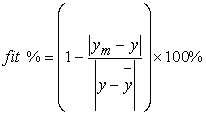(2)

where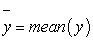.

The identified models are tabulated with their MSEs and fitting percentages in Table 1.

3.2. Model Uncertainty Estimation

Considering the transfer functions for the identified models tabulated in Table 1, the parametric uncertain model was built to be used in H robust controller design.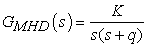(3)

The uncertain parameters of the model and their intervals are: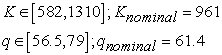The nominal model can be written as follows:(4)

4. Robust Controller Design

In this section, a novel approach to design a robust lead compensator based on Hrobust controller theory will be detailed. The overall philosophy in the design procedure presented here is to design a robust Hcontroller, then reduce its order maintaining its characteristics in the working frequency range, next it will be re-arranged to get the final compensator form.

4.1. System requirements

The required performance of the EMA system to be designed is a deadbeat response as shown in Table 2.

Consequently, the frequency domain system requirements may be derived from Table 2 as follows: The settling time constraint leads to minimum bandwidth 10 Hz. The overshoot constraint (< 1%) assigns the minimum damping ratio to 0.826, and the minimum phase margin should be 40 deg.

4.2. H∞ Robust Controller Design

H theory presents powerful frequency-domain framework for capturing control design requirements such as bandwidth, response speed, robust stability, and disturbance rejection . The EMA system in H framework is illustrated in Figure 3.

K is the robust controller, G: Motor and harmonic drive system, r: command reference signal, u: control signal, e: tracking error, and y: plant output. Define the error sensitivity function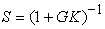, the control signal sensitivity function, and the complementary sensitivity function.

Robust performance constraint: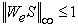Robust Stability constraint: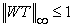Control signal (saturation) constraint:W, Wu and We are frequency dependent weighting functions.

The objective is to find controller K that stabilizes Gnom and satisfies H norm of a transfer matrix consisting of weighting functions is smaller than one, i.e.: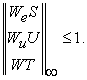This problem is called mixed sensitivity problem .

Here, we consider the multiplicative uncertainty to describe the deviation of the nominal model from the real system due to the ignored nonlinearities in real system. Assume GMHD is the models of the motor and harmonic drive, identified from the test sets tabulated in Table 1, and Gnom is the nominal model. Using the multiplicative uncertainty definition:(5)

where W(s) is the uncertainty weighting function, and Δ is an operator with infinity-norm less than unity , i.e.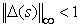, so(6)

By plotting the frequency response of, the multiplicative uncertainty weighting function can be extracted from the upper bound of different uncertainty frequency plots of the identified uncertain system; they are shown in Figure 4. The multiplicative uncertainty weighting function is approximated by: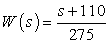which illustrates that robustness boundary provides 8 dB of gain below 30 rad/sec and suppress to more than -10 dB above 600 rad/sec. See Figure 5.

It may be concluded from Figure 4 that the uncertainty at low frequencies is small (about -7.5 dB), which allow robust control of the EMA system within this bandwidth.

The sensitivity weighting function is assigned to:which indicates sensitivity reduction of 100:1 (- 40 dB) for frequencies up to 1 rad/sec (i.e. at low freq. the closed loop should reject disturbance at the output by a factor of 100 to 1 or the steady state tracking errors due to step input should be less than 1%), as depicted in Figure 5.

Figure 5. Closed loop sensitivity, complementary sensitivity, robustness and performance functions with Hcontroller; simulation

The control signal sensitivity weighting function Wu (the actuator saturation weighting function) is chosen considering the physical limitation of the EMA. Thus, it can be considered to be a constant, so that the maximum expected input r (2 volt in this case) never saturates the actuator (saturation voltage 27 volt). Its value is estimated to be: Wu = 0.02.

The H controller, designed by Matlab and verifying H cost γ = 0.721, is:(7)

Since the H-norm of the closed-loop system is less than one, the condition:is satisfied in the given case. This may be checked by computing the sensitivity function of the closed-loop system and comparing it with the inverse of the performance weighting function. The result of the comparison is shown in Figure 5. It is seen that the sensitivity function lies below 1/We.

Similarly, the conditionmay be checked by computing the complementary sensitivity function of the closed-loop system and comparing it with the inverse of therobustness weighting function. The result of the comparison is shown in Figure 5, where the complementary sensitivity function lies beneath 1/W.

4.3. Compensator Derivation

Low order controllers are normally preferred over high order controllers in control system since they are computationally less demanding; easier to implement and they have higher reliability due to fewer things to go wrong in the hardware . The dc-gain of EMA system plays an important role in assessing its performance, for this reason it should be maintained. The 3rd order designed Hcontroller will be reduced in a manner to keep its characteristics in the working frequency range using balanced residualisation (singular perturbation approximation) method which gives best approximation at low frequencies .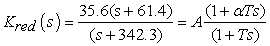(8)

which is a lead compensator with A = 35.6, α = 5.58, and T = 2.92 x 10-3. Figure 6 shows that the H and the reduced order controllers are almost identical in the range below 100 Hz which is sufficiently wider than our application's range (10 Hz). This controller can be easily realized using passive and active components consisting of resistors, capacitors, transistors, diodes and op-amps .

4.4. Compensator Realization

The lead compensator is realized by active components circuit shown in Figure 7.

The compensator transfer function is: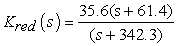(9)

From the circuit diagram shown in Figure 7, R1, R2 and C form the compensator network where as Rf and R0 form the compensator gain (A). The transfer function from the input voltage to the controller V1 to the output voltage V2 is: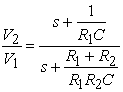(10)

Op-Amp: TL074, R1 = 162 KΩ, C = 0.1 μF and R2= 36 KΩ.The constant gain A = 35.6 is verified by the used compensator circuit in Figure 7; this is given by:4.5. Performance Validation of the Robust EMA with H∞ derived Compensator

Table 3 illustrates the time response values for the H controller and the derived lead compensator, which clarify that there is no considerable performance retreat.

Table 3. Characteristics of Robust EMAs with H∞Controller and Derived Compensator

The step responses and bode plots of the uncertain closed loop EMA syetem with the derived lead compensator are depicted in Figure 8 and Figure 9, respectively.

The new designed EMA system with lead compensator derived from the Hcontroller, in addition to its robustness to parametric uncertainties, has almost the same dynamics of the Hcontroller and the simplicity advantages of compensators.

In order to verify that other system requirements in Table 2 are met with the derived compensator, the margins and bandwidths were tabulated in Table 4.

Table 4. Margins of Uncertain Plants with the Compensator

It is noted that system requirements are verified.

The control signal may be checked so that the actuator will not be saturated during operation. The control signals to the actuator corresponding to maximum input command (2 volts) with the designed robust controllers were plotted (as illustrated in Figure 10).

Finally, the performance of the robust EMA with H derived compensator was compared with the original classic controller EMA performance. It proves, in addition to its robustness to parametric uncertainties, better dynamic performance, as shown in Figure 11.

Figure 11. Measured step for original and robust EMA systems; experiment

5. Conclusion

An approach to design robust compensator, based on H theory, was applied on an EMA harmonic drive system. It leaded to a robust EMA system which is, in addition to its robustness, simple and easily implementable.

The robust controller design and validation procedure is studied by simulation and experiments, and its effectiveness is proven by comparing the performance of compensator with the robust H controller and the original EMA system with classic controller. The comparison has demonstrated the effectiveness of the proposed robust EMA system with lead compensator.

The proposed approach applied on the EMA system, may be applied on other control systems operating over a relatively low range of frequencies.

References

  Ristanović, M., Ćojbašić, Ž. and Lazić, D., “Intelligent control of DC motor driven electromechanical fin actuator,” J. Control Engineering Practice, 20 (6). 610-617. Mar. 2012.In article CrossRef  Liscouët, J., MaréJ. C. and Budinger, M., “An integrated methodology for the preliminary design of highly reliable electromechanical actuators: Search for architecture solutions,” Aerospace Science and Technology, 22 (1). 9-18. Oct-Nov. 2012.In article CrossRef  Walter, E., Pronzato, L. and Norton, J., Identification of Parametric Model from Experimental Data, Springer, GB, 1997.In article  Lu, H., Li, Y. and Zhu, C., “Robust synthesized control of electromechanical actuator for thrust vector system in spacecraft,” J. Computers and Mathematics with Applications, 64 (5). 699-708. Sept. 2012.In article CrossRef  Toscano, R., “A simple robust PI/PID controller design via numerical optimization approach,” J. of process control, 15 (1). 81-88. Feb. 2005.In article CrossRef  ValérioD. and , J., “Tuning of Fractional Controllers Minimising H2 and H∞ Norms,” Acta Polytechnica Hungarica, 3 (4). 55-70. 2006.In article  Yogesh, V. H., Gupta, J. R. P. and Choudhury, R., “Kharitonov’s Theorem and Routh Criterion for Stability Margin of Interval Systems,” International Journal of Control, Automation, and Systems, 8 (3). 647-654. June 2010.In article CrossRef  Saadaoui, K. and Özgüler, A. B., “A new method for the computation of all stabilizing controllers of a given order,” International Journal of Control, 78 (1). 14-28. Jan. 2005.In article CrossRef  Tantaris, R. N., Keel, L. H. and Bhattacharyya, S. P., “Stabilization of discrete-time systems by first-order controllers,” IEEE Trans. Automat Control, 48 (5). 858-861. May 2003.In article CrossRef  Tan, N., “Computation of stabilizing PI-PD controllers,” International Journal of Control, Automation, and Systems, 7 (2). 175-184. April 2009.In article CrossRef  Yeroglu, C. and Tan, N., “Design of Robust PI Controller for Vehicle Suspension System,” Journal of Electrical Engineering & Technology, 3 (1). 135-142. 2008.In article CrossRef  Huang, Y. J. and Wang, Y.-J., “Robust PID tuning strategy for uncertain plants based on the Kharitonov theorem,” ISA Transactions, 39 (4). 419-431. Sept. 2000.In article CrossRef  Li, X. H., Yu, H.B., Yuan, M.Z. and Wang, J., “Design of robust optimal proportional-integral-derivative controller based on new interval polynomial stability criterion and Lyapunov theorem in the multiple parameters’ perturbations circumstance,” IET Control Theory Appl., 4 (11). 2427-2440. Nov. 2010.In article CrossRef  Rigatos, G. and Siano, P., “Design of robust electric power system stabilizers using Kharitonov’s theorem,” Mathematics and Computers in Simulation, 82 (1). 181-191. Sept. 2011.In article CrossRef  Yoo, C.-H., Lee, Y.-C. and Lee, S.-Y., “A Robust Controller for an Electro-Mechanical Fin Actuator,” in Proceeding of the American Control Conference, Boston, Massachusetts, 5, June 30-July 2, 2004, 4010-4015.In article  Benderradji, H., Chrifi-Alaoui, L., Mahieddine-Mahmoud S. and Makouf, A., “Robust Control of Induction Motor with H∞ Theory based on Loopshaping,” Journal of Electrical Engineering & Technology, 6 (2). 226-232. 2011.In article CrossRef  Raafat, S. M., Akmeliawati, R. and Abdulljabaar, I., “Robust H∞ Controller for High Precision Positioning System, Design, Analysis, and Implementation,” Intelligent Control and Automation, 3 (3). 262-273. Aug. 2012.In article CrossRef  Ljung, L., System Identification: Theory for the User, 2nd edition, Prentice-Hal PTR, Upper Saddle River, NJ, 1999.In article  Bhattacharyya, S. P., Chapellat, H. and Keel, L. H., Robust Control: The Parametric Approach, Prentice Hall, 1995.In article  Tavakoli, M., Taghirad, H. D., and Abrishamchian, M., “Identification and Robust H∞ Control of the Rotational/Translational Actuator System,” International Journal of Control, Automation, and Systems, 3 (3). 387-396. Sept. 2005.In article  Lim, K. B., Cox, D. E., Balas, G. J. and Juang, J.-N., “Validation of an Experimentally Derived Uncertainty Model,” Journal of Guidance, Control, and Dynamics, 21 (3). 485-492. May-June 1998.In article CrossRef  Zhou, K., Doyle, J. C. and Glover, K., Robust and Optimal Control, Prentice Hall, Englewood Cliffs, New Jersey, 1996.In article  Oh, D. C., Bang, K. H. and Park, H. B., “Controller order reduction using singular perturbation approximation,” , 33 (6). 1203-1207. June 1997.In article CrossRef  Kuo, C. B., Automatic Control System, 5th edition. Prentice Hall of Indian Private ltd, New Delhi, 1989.In article